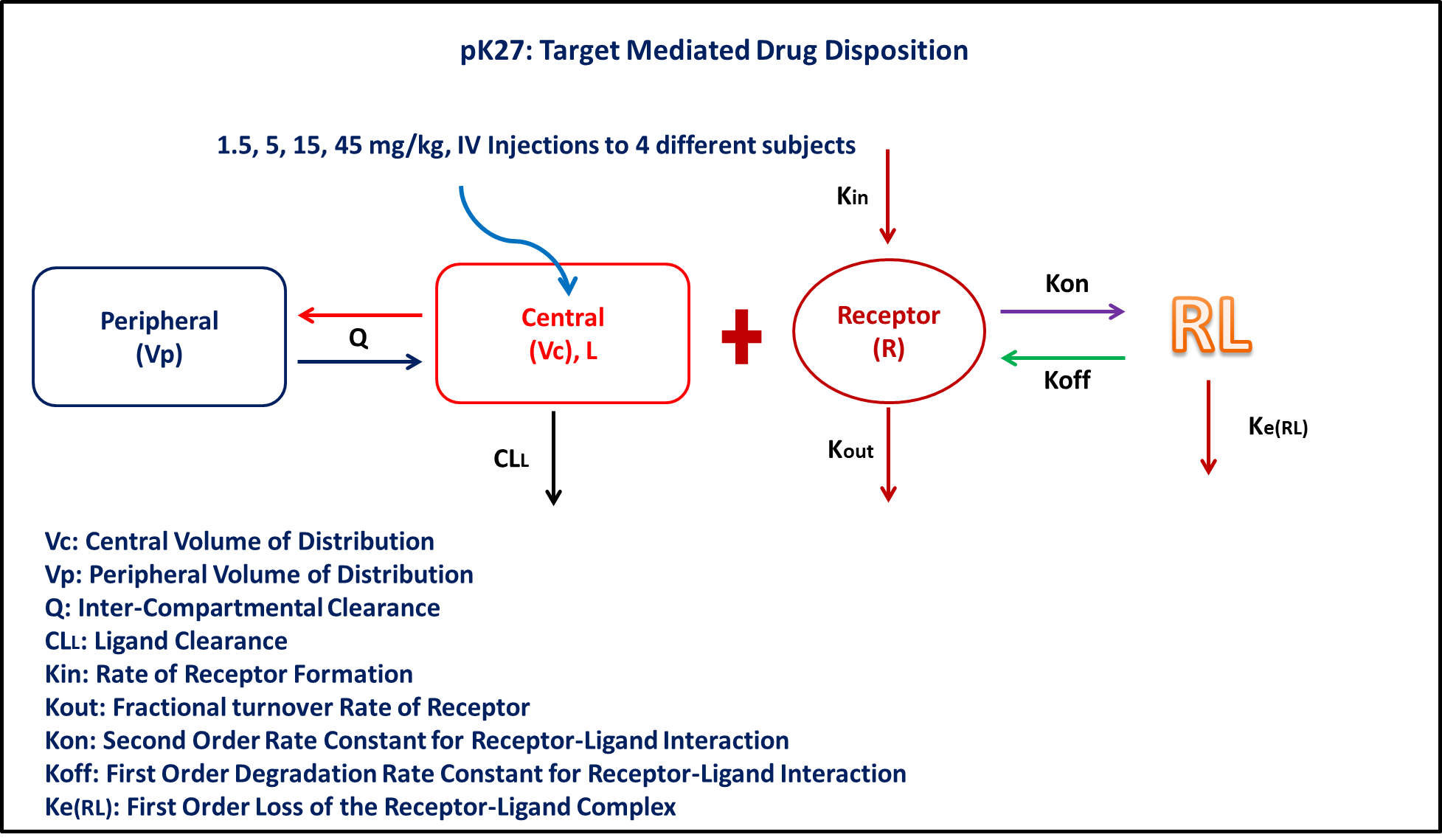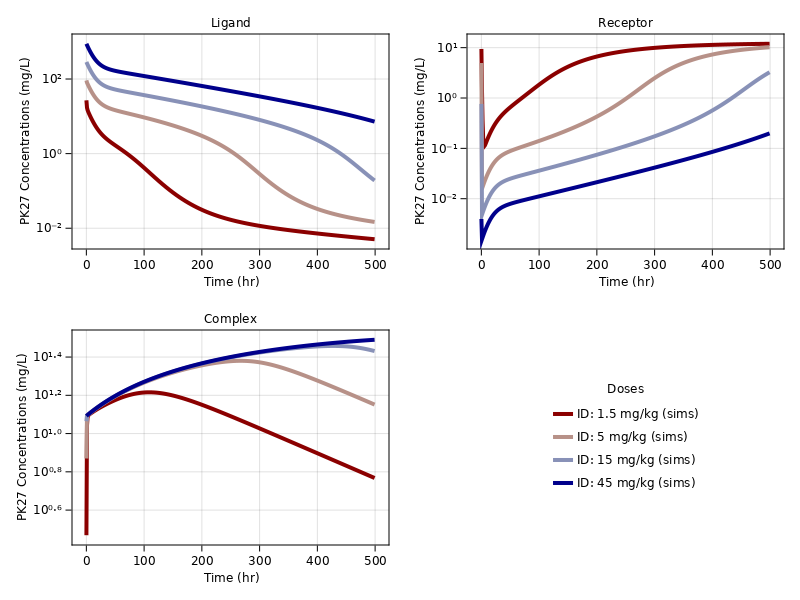# Exercise PK-27 - Target Mediated Drug Disposition

### Background

• Structural model - Target Mediated Drug Disposition Model (TMDD)

• Route of administration - IV-Bolus

• Dosage Regimen - 1.5, 5, 15, 45 mg/kg administered after complete washout

• Number of Subjects - 4### Learning Outcome

• To fit a full TMDD model with data from only ligand, ligand and target, target and ligand-target complex

• Write a differential equation for a full TMDD model

### Objective

• The objective of this exercise is to simulate from a TMDD model

### Libraries

Call the "necessary" libraries to get started

using Random
using Pumas
using PumasUtilities
using CairoMakie


### Model

pk_27           = @model begin
desc        = "Target Mediated Drug Disposition Model"
timeu       = u"hr"
end

@param begin
"Clearance of central compartment (L/kg/hr)"
tvcl        ∈ RealDomain(lower=0)
"Second oder on rate of ligand (L/mg/hr)"
tvkon       ∈ RealDomain(lower=0)
"First order off rate of ligand (1/hr)"
tvkoff      ∈ RealDomain(lower=0)
"Volume of Peripheral Compartment (L/kg)"
tvvp        ∈ RealDomain(lower=0)
"Inter-compartmental clearance (L/kg/hr)"
tvq         ∈ RealDomain(lower=0)
"Zero order receptor synthesis process (mg/L/hr)"
tvkin       ∈ RealDomain(lower=0)
"First order receptor degenration process (1/hr)"
tvkout      ∈ RealDomain(lower=0)
"First order elimination of complex (1/hr)"
tvkerl      ∈ RealDomain(lower=0)
"Volume of Central Compartment (L/kg)"
tvvc        ∈ RealDomain(lower=0)
Ω           ∈ PDiagDomain(9)
"Proportional RUV - Plasma"
σ²_prop_cp  ∈ RealDomain(lower=0)
"Proportional RUV - Receptor"
σ²_prop_rec ∈ RealDomain(lower=0)
"Proportional RUV - Complex"
σ²_prop_com ∈ RealDomain(lower=0)
end

@random begin
η           ~ MvNormal(Ω)
end

@pre begin
Cl          = tvcl * exp(η)
Kon         = tvkon * exp(η)
Koff        = tvkoff * exp(η)
Vp          = tvvp * exp(η)
Q           = tvq * exp(η)
Kin         = tvkin * exp(η)
Kout        = tvkout * exp(η)
Kerl        = tvkerl * exp(η)
Vc          = tvvc * exp(η)
end

@dosecontrol begin
Vc_          = tvvc * exp(η)
bioav       = (Central = 1/Vc_,)
end

@init begin
Receptor    = Kin/Kout
end

@dynamics begin
Central'    = -(Cl/Vc)*Central - (Q/Vc)*Central + (Q/Vp)*Peripheral - Kon*Receptor*Central + Koff*Complex
Peripheral' = (Q/Vc)*Central - (Q/Vp)*Peripheral
Receptor'   = Kin - Kout*Receptor - Kon*Receptor*Central + Koff*Complex
Complex'    = Kon*Receptor*Central - Koff*Complex - Kerl*Complex
end

@derived begin
cp          = @. Central
"""
Observed Concentration - Plasma (mg/L)
"""
dv_cp       = @. Normal(cp, sqrt(cp^2*σ²_prop_cp))
rec         = @. Receptor
"""
Observed Concentration - Receptor (mg/L)
"""
dv_rec      = @. Normal(rec, sqrt(rec^2*σ²_prop_rec))
com         = @. Complex
"""
Observed Concentration - Complex (mg/L)
"""
dv_com      = @. Normal(com, sqrt(com^2*σ²_prop_com))
end
end

PumasModel
Parameters: tvcl, tvkon, tvkoff, tvvp, tvq, tvkin, tvkout, tvkerl, tvvc,
Ω, σ²_prop_cp, σ²_prop_rec, σ²_prop_com
Random effects: η
Covariates:
Dynamical variables: Central, Peripheral, Receptor, Complex
Derived: cp, dv_cp, rec, dv_rec, com, dv_com
Observed: cp, dv_cp, rec, dv_rec, com, dv_com


### Parameters

The parameters are as given below. tv represents the typical value for parameters.

• $Cl$ - Clearance of central compartment (L/kg/hr)

• $Kon$ - Second oder on rate of ligand (L/mg/hr)

• $Koff$ - First order off rate of ligand (1/hr)

• $Vp$ - Volume of Peripheral Compartment (L/kg)

• $Q$ - Inter-compartmental clearance (L/kg/hr)

• $Kin$ - Zero order receptor synthesis process (mg/L/hr)

• $Kout$ - First order receptor degeneration process (1/hr)

• $Kerl$ - First order elimination of complex (1/hr)

• $Vc$ - Volume of Central Compartment (L/kg)

param = ( tvcl        = 0.001,
tvkon       = 0.096,
tvkoff      = 0.001,
tvvp        = 0.100,
tvq         = 0.003,
tvkin       = 0.11,
tvkout      = 0.0089,
tvkerl      = 0.003,
tvvc        = 0.05,
Ω           = Diagonal([0.0,0.0,0.0,0.0,0.0,0.0,0.0,0.0,0.0]),
σ²_prop_cp  = 0.02,
σ²_prop_rec = 0.012,
σ²_prop_com = 0.015)

(tvcl = 0.001, tvkon = 0.096, tvkoff = 0.001, tvvp = 0.1, tvq = 0.003, tvki
n = 0.11, tvkout = 0.0089, tvkerl = 0.003, tvvc = 0.05, Ω = [0.0 0.0 … 0.0
0.0; 0.0 0.0 … 0.0 0.0; … ; 0.0 0.0 … 0.0 0.0; 0.0 0.0 … 0.0 0.0], σ²_prop_
cp = 0.02, σ²_prop_rec = 0.012, σ²_prop_com = 0.015)


### Dosage Regimen

• Single dose of 1.5 mg/kg was administered as IV-Bolus at time=0

• Single dose of 5 mg/kg was administered as IV-Bolus at time=0

• Single dose of 15 mg/kg was administered as IV-Bolus at time=0

• Single dose of 45 mg/kg was administered as IV-Bolus at time=0

dose = [1.5, 5, 15, 45]
ids  = ["1.5 mg/kg", "5 mg/kg", "15 mg/kg", "45 mg/kg"]
dose_ind(x) = DosageRegimen(dose[x], time = 0, cmt = 1)

pop4_sub = map(i -> Subject(id = ids[i], events = dose_ind(i),
observations = (cp = nothing, rec = nothing, com = nothing)),
1:length(ids))

Population
Subjects: 4
Observations: cp, rec, com


### Simulation

Random.seed!(123)
sim_pop4 = simobs(pk_27, pop4_sub, param, obstimes = 0.1:1:500)


### Visualization

figure = Figure(; fontsize = 12)
a1, p1 = sim_plot(figure[1,1], pk_27, sim_pop4,
observations = :cp,
color = :redsblues,
linewidth = 4,
axis = (title = "Ligand",
xlabel = "Time (hr)",
ylabel = "PK27 Concentrations (mg/L)",
xticks = 0:100:500, yscale = log10))

a2, p2 = sim_plot(figure[1,2], pk_27, sim_pop4,
observations = :rec,
color = :redsblues,
linewidth = 4,
axis = (title = "Receptor",
xlabel = "Time (hr)",
ylabel = "PK27 Concentrations (mg/L)",
xticks = 0:100:500, yscale = log10))

a3, p3 = sim_plot(figure[2,1], pk_27, sim_pop4,
observations = :com,
color = :redsblues,
linewidth = 4,
axis = (title = "Complex",
xlabel = "Time (hr)",
ylabel = "PK27 Concentrations (mg/L)",
xticks = 0:100:500, yscale = log10))
Legend(figure[2, 2], a3, "Doses", tellwidth = false, framevisible = false)
figure